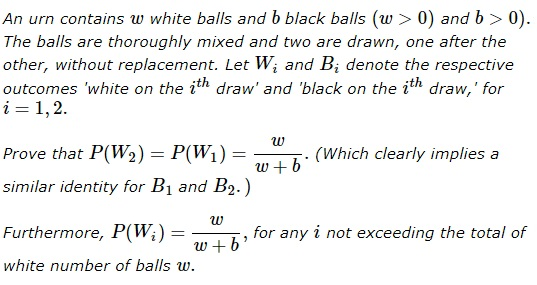# Balls of Two Colors

### Problem### Solution 1

We must remember the formula for the total probability:

$P(W_{2}) = P(W_{2}|W_{1})\cdot P(W_{1}) + P(W_{2}|B_{1})\cdot P(B_{1}),$

from which

\begin{align}\displaystyle P(W_{2}) &= \frac{w - 1}{b + w - 1}\cdot \frac{w}{b + w} + \frac{w}{b + w - 1}\cdot \frac{b}{b + w} \\ &=\frac{w\cdot (w - 1 + b)}{(b + w - 1)\cdot (b + w)}, \end{align}

which is simplified to $\displaystyle\frac{w}{w+b}.$

The wonderful thing here is that the initial probability $\displaystyle\frac{w}{w+b}$ is being maintained even though the balls are not returned to the urn.

### Solution 2

$\displaystyle P (W_1)=\frac{w}{b+w},$ $\displaystyle P (B_1)=\frac{b}{b+w}.$ And further,

\displaystyle\begin{align}P (W_2)&=P(B_1) \frac{ w}{b+w-1}+P(W_1)\frac{ (w-1) }{b+w-1}\\ &=\frac{(w-2) \left(\frac{w}{b+w}\right)^2}{b+w-2}+\frac{(w-1) w \left(1-\frac{w}{b+w}\right)}{(b+w-2) (b+w)}\\ &\qquad\qquad\qquad+\frac{(w-1) w \left(1-\frac{w}{b+w}\right)}{(b+w-2) (b+w)}+\frac{w \left(1-\frac{w}{b+w}\right)^2}{b+w-2}\\ &=\frac{w}{b+w}. \end{align}

More generally

$\displaystyle P( W_n)=\sum _{k=0}^n \frac{\left(1-\frac{w}{b+w}\right)^k \binom{n}{k} (k-n+w) \left(\frac{w}{b+w}\right)^{n-k}}{b-n+w}=\frac{w}{b+w}.$

### Solution 3

If you empty the urn $1$ ball at a time you will generate a sequence of length $n=w+b.$ By exchangeability, each sequence is equally likely. What is the probability that at the $i^{th}$ draw you draw $w$? It's the number of unique sequences with $w$ in the $i^{th}$ position, $\displaystyle \binom{n-1}{w-1}$, divided by the number of unique sequences $\displaystyle \binom{n}{w}$, i.e.

\displaystyle \begin{align} \frac\binom{n-1}{w-1}}\binom{n}{w}}&=\frac\frac{(n-1)!}{(w-1)!(n-w)!}}\frac{n!}{w!(n-w)!}}\\ &=\frac{(n-1)!w!(n-w)!}{n!(w-1)!(n-w)!}\\ &=\frac{w}{n}\\ &=\frac{w}{w+b} \end{align

### Acknowledment

The problem and Solution 1 came from the references below. Solution 2 is by N. N. Taleb; Solution 3 is by Joshua B. Miller.

### References

1. Ruma Falk, Understanding Probability and Statistics, A K Peters, 1993
2. Paul Nahin, Will You Be Alive In 10 Years From Now?, Princeton University Press, 2013 (Introduction)